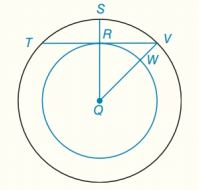Chapter 6.CT, Problem 8CTElementary Geometry For College St...

7th Edition
Alexander + 2 others
ISBN: 9781337614085

Solutions

Chapter
SectionElementary Geometry For College St...

7th Edition
Alexander + 2 others
ISBN: 9781337614085
Textbook Problem

a) Because point Q is their common center, these circles are known as                                                         _ circles.b) If R Q = 3 and Q V = 5 , find the length of chord T V ¯ which is also a tangent to the inner circle.To determine

(a)

To fill:

The blank in the statement “Because point Q is their common center, these circles are known as _ circles”.

Explanation

Definition used:

Concentric circles are coplanar circles that have a common center.

Given:

Q is the common center for two circles.

Approach:

Clearly, Q is the common center for two circles

To determine

(b)

To find:

The length of chord TV¯ which is tangent to the inner circle.

Still sussing out bartleby?

Check out a sample textbook solution.

See a sample solution

The Solution to Your Study Problems

Bartleby provides explanations to thousands of textbook problems written by our experts, many with advanced degrees!

Get Started

Multiply: 1520320

Elementary Technical Mathematics

13. Find the relative maxima, relative minima, and points of inflection of the graph of . .

Mathematical Applications for the Management, Life, and Social Sciences

In Exercises 1 and 2, simplify the expression. 4(10)75

Calculus: An Applied Approach (MindTap Course List)

The general solution to dydt=ky is: a) y(t) = y(0)ekt b) y(t) = y(k)et c) y(t) = y(t)ek d) y(t) = ey(0)kt

Study Guide for Stewart's Single Variable Calculus: Early Transcendentals, 8th

Suppose that A and B are mutually exclusive events and that P(AB)0. What is P(AAB)?

Finite Mathematics for the Managerial, Life, and Social Sciences

Using for |x| < 1,

Study Guide for Stewart's Multivariable Calculus, 8th